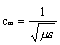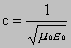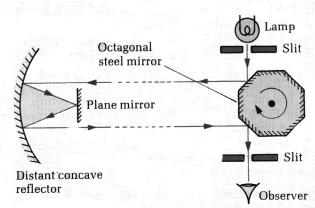9.2.3 Electromagnetic waves

In 1831 Michael Faraday discovered electromagnetic induction. This is where a changing magnetic field creates an electric field. The two fields are at right angles to each other and an electrical field is created whenever there is a changing magnetic field.

In 1862 James Clerk Maxwell devised a theory linking electricity and magnetism together, using Faraday’s discoveries. Faraday had found that a magnetic field was created around a wire carrying an electrical current, and that a changing magnetic field could create an electrical current (the principle of electromagnetic induction).

Maxwell suggested that a changing magnetic field could create a changing electrical field, and that a changing electrical field could produce a changing magnetic field. Maxwell made this suggestion because if it were proved to be the case then the laws governing electricity and magnetism became both simple and symmetrical. It was difficult to provide evidence for Maxwell’s assumption because it was very difficult to detect a weak magnetic field at the time but one consequence of Maxwell’s prediction was that any electric field produced by a changing magnetic field must itself produce a magnetic field, and vice versa, ie. one effect must always be linked to the other.

This led Maxwell to predict that an oscillating electric charge would produce an electromagnetic wave consisting of a fluctuating electric field accompanied by a fluctuating magnetic field of the same frequency and in phase with it. Both fields would vary sinusoidally at right angles to each other and would oscillate at right angles to the direction of travel of the wave (ie. transverse) but no medium would be required for the wave to travel.

Maxwell also showed that the speed of these electromagnetic waves in any medium would be given byand in a vacuum bywhere m0 and e0  are the permeability and permittivity of free space respectively.

This equation gave a value for the speed of electromagnetic waves which was in very close agreement with the measured value for the speed of light waves.

It became accepted within the scientific community that light was an electromagnetic wave.

## Michelson’s measurement of the speed of light

follow this link the view an animation and have a go at some calculationsThe octagonal mirror is capable of being rotated at high speed. As the speed of rotation increases the image first disappears and then reappears at a certain frequency of rotation, f. Increased rotation makes the image disappear but it reappears at frequencies 2f, 3f, 4f etc. The reason why the image appears at frequency f is because in the time it takes for light to travel from the lamp to the observer the mirror has rotated through exactly 1/8 of a rotation. The trip time is exactly equal to 1/8f. If the trip distance is D then the speed of light is 8fD.

Michelson made a large number of measurements of the speed of light. A plot of the number of observations against the measured speed indicated that the likely value was 299 774 kms-1 which is very close to the currently agreed value of 299 793 kms-1.

## Hertz’s discovery of radio waves

By its magic the human voice may be projected around the earth in less time than it takes to pronounce the word”radio”

Archer.  History of radio to 1926.

In 1888 Heinrich Hertz produced a spark between the terminals of an induction coil (similar to a transformer but using dc to provide a pulse). A short distance away a spark occurred in a gap in another isolated loop of wire. Something had passed from the spark gap in the induction coil – the transmitter- to the spark gap in the loop – the receiver.

Hertz rapidly determined the properties of this new radiation and he showed that

·         the radiation travelled in straight lines and could produce phenomena similar to diffraction

·         the radiation was plane polarised ie. it consisted of transverse vibrations

·         the radiation obeyed the law of reflection

·         the radiation could be refracted

Hertz knew that the newly discovered waves were reflected by metals and he measured the speed of these waves by setting up a stationary wave and measuring the distance between adjacent nodes. The velocity was found to be approximately that expected for electromagnetic waves.

Some things to do or consider and discuss

1.       Explain diagrammatically how the corpuscular theory and the wave theory explain reflection.

2.       A theory is required before experiments can be undertaken.

3.       Experimental data is conclusive proof about a theory.

4.       Construct a table to show which properties of light can be explained by the wave theory and which by the particle theory.

5.       Remind yourself of the electromagnetic spectrum by using your textbooks.

6.       Use Maxwell’s equation to calculate a value for the speed of electromagnetic waves.

7.       Explain how polaroid filters demonstrate the transverse nature of light.

next